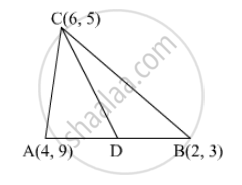Advertisement Remove all ads

# If A(4, 9), B(2, 3) and C(6, 5) are the vertices of ∆ABC, then the length of median through C is - Mathematics

MCQ

If A(4, 9), B(2, 3) and C(6, 5) are the vertices of ∆ABC, then the length of median through C is

#### Options

• 5 units

• $\sqrt{10}$ units

• 25 units

•  10 units

Advertisement Remove all ads

#### Solution

It is given that A(4, 9), B(2, 3) and C(6, 5) are the vertices of ∆ABC.Let CD be the median of ∆ABC through C. Then, D is the mid-point of AB.

Using mid-point formula, we get

Coordinates of D = $\left( \frac{4 + 2}{2}, \frac{9 + 3}{2} \right) = \left( \frac{6}{2}, \frac{12}{2} \right) = \left( 3, 6 \right)$

∴ Length of the median, AD

$= \sqrt{\left( 6 - 3 \right)^2 + \left( 5 - 6 \right)^2} \left( \text{ Using distance formula } \right)$
$= \sqrt{3^2 + \left( - 1 \right)^2}$
$= \sqrt{10} \text{ units }$

Thus, the length of the required median is $\sqrt{10}$ units.

Is there an error in this question or solution?
Advertisement Remove all ads

#### APPEARS IN

RD Sharma Class 10 Maths
Chapter 6 Co-Ordinate Geometry
Q 45 | Page 66
Advertisement Remove all ads

#### Video TutorialsVIEW ALL 

Advertisement Remove all ads
Share
Notifications

View all notifications

Forgot password?